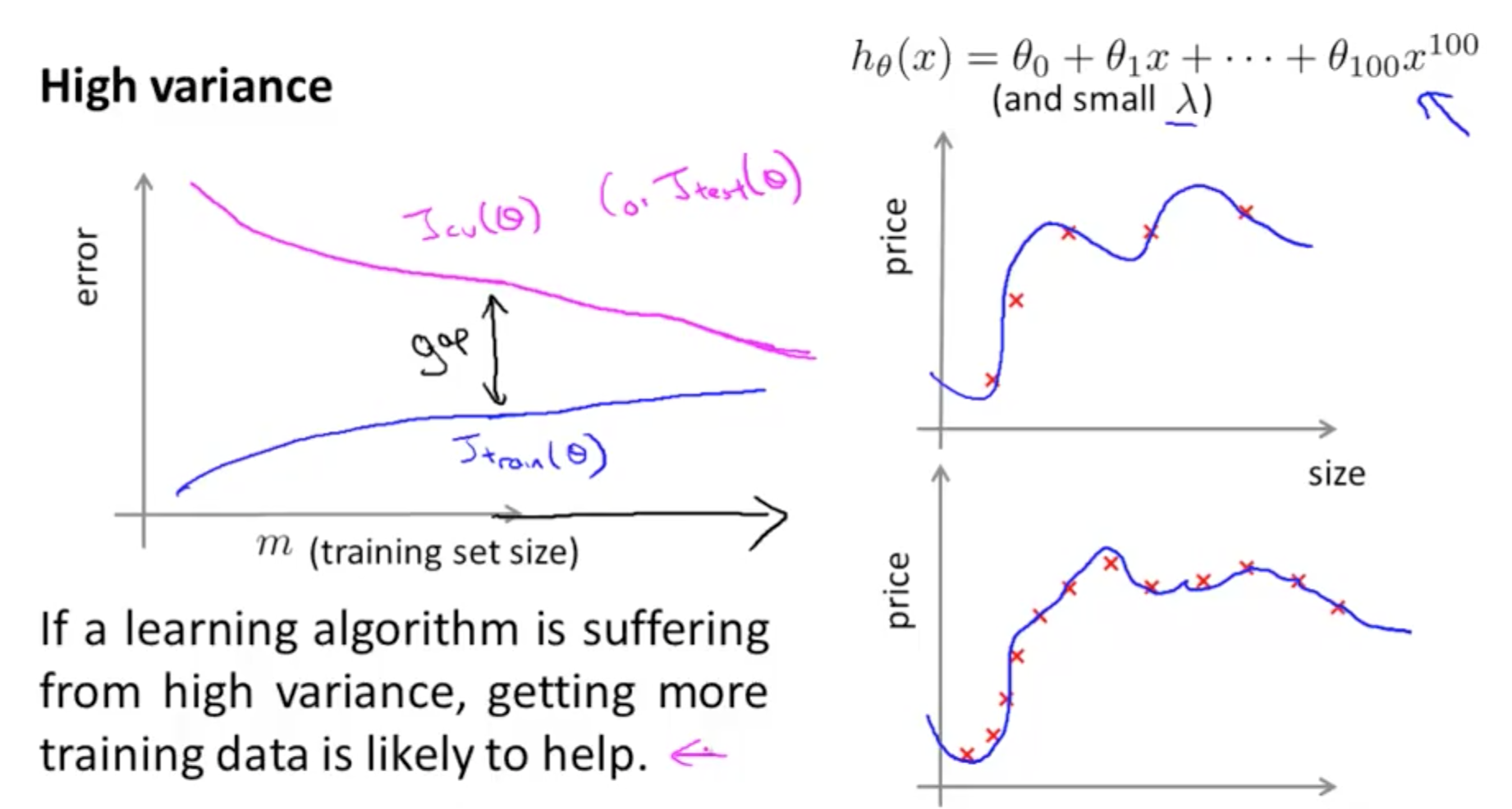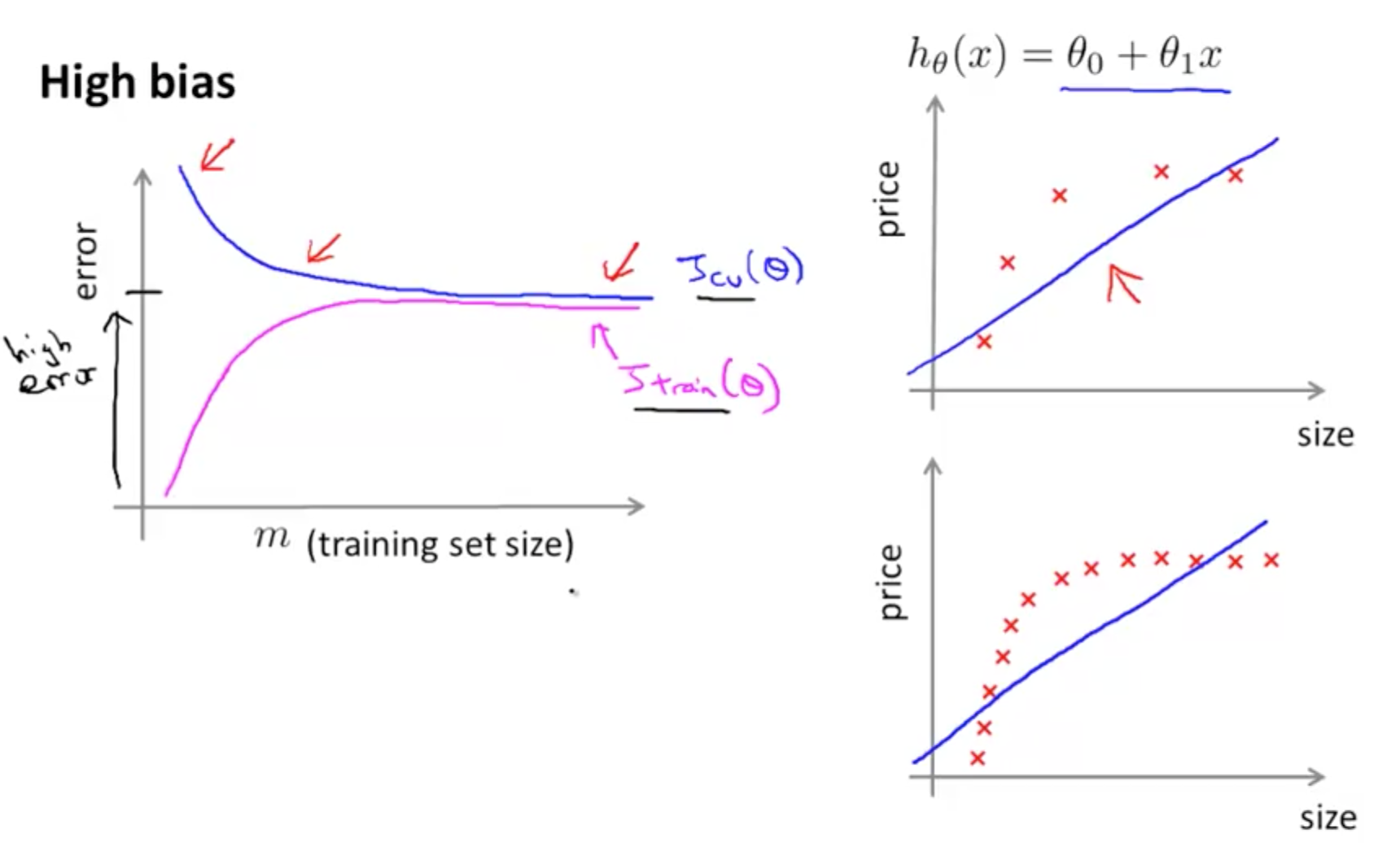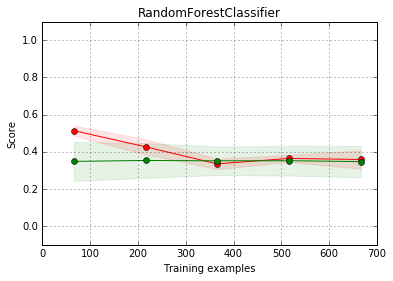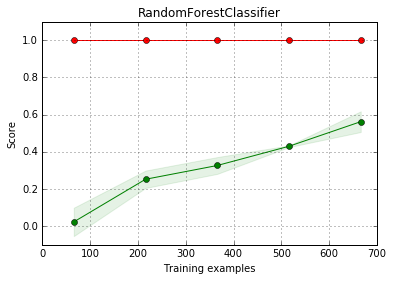Evaluate bias and variance with a learning curve
Learning Curve

Learning Curve Theory

• Graph that compares the performance of a model on training and testing data over a varying number of training instances
• We should generally see performance improve as the number of training points increases
• When we separate training and testing sets and graph them individually
• We can get an idea of how well the model can generalize to new data
• Learning curve allows us to verify when a model has learning as much as it can about the data
• When it occurs
1. The performances on the training and testing sets reach a plateau
2. There is a consistent gap between the two error rates
• The key is to find the sweet spot that minimizes bias and variance by finding the right level of model complexity
• Of course with more data any model can improve, and different models may be optimal
• For a more in-depth theoretical coverage of learning curves, you can view a guide by Andrew Ng that I have compiled here

Types of learning curves

• Bad Learning Curve: High Bias
• When training and testing errors converge and are high
• No matter how much data we feed the model, the model cannot represent the underlying relationship and has high systematic errors
• Poor fit
• Poor generalization
• Bad Learning Curve: High Variance
• When there is a large gap between the errors
• Require data to improve
• Can simplify the model with fewer or less complex features
• Ideal Learning Curve
• Model that generalizes to new data
• Testing and training learning curves converge at similar values
• Smaller the gap, the better our model generalizesExample 1: High Bias

• In this example, you'll see that we'll be using a linear learner on quadratic data
• The result is that we've high bias
• We'll have a low score (high error)
In :
# imports
from sklearn.linear_model import LinearRegression
from sklearn.learning_curve import learning_curve
import matplotlib.pyplot as plt
from sklearn.metrics import explained_variance_score, make_scorer
from sklearn.cross_validation import KFold
import numpy as np

In :
size = 1000
cv = KFold(size, shuffle=True)


Create X array

In :
#np.reshape(old_shape, new_shape)

# new array (-1, 1)
# -1 implies to take shape from original, hence 1000
# this creates a 1000 x 1 array
X = np.reshape(np.random.normal(scale=2,size=size),(-1,1))
X.shape

Out:
(1000, 1)
In :
# np.random.normal(scale=2,size=size) creates a 1000 x 1 matrix
# scale=2 is the standard deviation of the distribution
np.random.normal(scale=2,size=size).shape

Out:
(1000,)

Create y array

In :
y = np.array([[1 - 2*x +x**2] for x in X])
y.shape

Out:
(1000, 1)

Plot learning curve

In :
def plot_curve():
# instantiate
lg = LinearRegression()

# fit
lg.fit(X, y)

"""
Generate a simple plot of the test and traning learning curve.

Parameters
----------
estimator : object type that implements the "fit" and "predict" methods
An object of that type which is cloned for each validation.

title : string
Title for the chart.

X : array-like, shape (n_samples, n_features)
Training vector, where n_samples is the number of samples and
n_features is the number of features.

y : array-like, shape (n_samples) or (n_samples, n_features), optional
Target relative to X for classification or regression;
None for unsupervised learning.

ylim : tuple, shape (ymin, ymax), optional
Defines minimum and maximum yvalues plotted.

cv : integer, cross-validation generator, optional
If an integer is passed, it is the number of folds (defaults to 3).
Specific cross-validation objects can be passed, see
sklearn.cross_validation module for the list of possible objects

n_jobs : integer, optional
Number of jobs to run in parallel (default 1).

x1 = np.linspace(0, 10, 8, endpoint=True) produces
8 evenly spaced points in the range 0 to 10
"""

train_sizes, train_scores, test_scores = learning_curve(lg, X, y, n_jobs=-1, cv=cv, train_sizes=np.linspace(.1, 1.0, 5), verbose=0)

train_scores_mean = np.mean(train_scores, axis=1)
train_scores_std = np.std(train_scores, axis=1)
test_scores_mean = np.mean(test_scores, axis=1)
test_scores_std = np.std(test_scores, axis=1)

plt.figure()
plt.title("RandomForestClassifier")
plt.legend(loc="best")
plt.xlabel("Training examples")
plt.ylabel("Score")
plt.gca().invert_yaxis()

# box-like grid
plt.grid()

# plot the std deviation as a transparent range at each training set size
plt.fill_between(train_sizes, train_scores_mean - train_scores_std, train_scores_mean + train_scores_std, alpha=0.1, color="r")
plt.fill_between(train_sizes, test_scores_mean - test_scores_std, test_scores_mean + test_scores_std, alpha=0.1, color="g")

# plot the average training and test score lines at each training set size
plt.plot(train_sizes, train_scores_mean, 'o-', color="r", label="Training score")
plt.plot(train_sizes, test_scores_mean, 'o-', color="g", label="Cross-validation score")

# sizes the window for readability and displays the plot
# shows error from 0 to 1.1
plt.ylim(-.1,1.1)
plt.show()

In :
%matplotlib inline
plot_curve()Compared to the theory we covered, here our y-axis is 'score', not 'error', so the higher the score, the better the performance of the model.

• Training score (red line) decreases and plateau
• Indicates underfitting
• High bias
• Cross-validation score (green line) stagnating throughout
• Unable to learn from data
• Low scores (high errors)
• Should tweak model (perhaps increase model complexity)

Example 2: High Variance

• Noisy data and complex model

There're no inline notes here as the code is exactly the same as above and are already well explained.

In :
from sklearn.tree import DecisionTreeRegressor

X = np.round(np.reshape(np.random.normal(scale=5,size=2*size),(-1,2)),2)
y = np.array([[np.sin(x+np.sin(x))] for x in X])

def plot_curve():
# instantiate
dt = DecisionTreeRegressor()

# fit
dt.fit(X, y)

"""
Generate a simple plot of the test and traning learning curve.

Parameters
----------
estimator : object type that implements the "fit" and "predict" methods
An object of that type which is cloned for each validation.

title : string
Title for the chart.

X : array-like, shape (n_samples, n_features)
Training vector, where n_samples is the number of samples and
n_features is the number of features.

y : array-like, shape (n_samples) or (n_samples, n_features), optional
Target relative to X for classification or regression;
None for unsupervised learning.

ylim : tuple, shape (ymin, ymax), optional
Defines minimum and maximum yvalues plotted.

cv : integer, cross-validation generator, optional
If an integer is passed, it is the number of folds (defaults to 3).
Specific cross-validation objects can be passed, see
sklearn.cross_validation module for the list of possible objects

n_jobs : integer, optional
Number of jobs to run in parallel (default 1).

x1 = np.linspace(0, 10, 8, endpoint=True) produces
8 evenly spaced points in the range 0 to 10
"""

train_sizes, train_scores, test_scores = learning_curve(dt, X, y, n_jobs=-1, cv=cv, train_sizes=np.linspace(.1, 1.0, 5), verbose=0)

train_scores_mean = np.mean(train_scores, axis=1)
train_scores_std = np.std(train_scores, axis=1)
test_scores_mean = np.mean(test_scores, axis=1)
test_scores_std = np.std(test_scores, axis=1)

plt.figure()
plt.title("RandomForestClassifier")
plt.legend(loc="best")
plt.xlabel("Training examples")
plt.ylabel("Score")
plt.gca().invert_yaxis()

# box-like grid
plt.grid()

# plot the std deviation as a transparent range at each training set size
plt.fill_between(train_sizes, train_scores_mean - train_scores_std, train_scores_mean + train_scores_std, alpha=0.1, color="r")
plt.fill_between(train_sizes, test_scores_mean - test_scores_std, test_scores_mean + test_scores_std, alpha=0.1, color="g")

# plot the average training and test score lines at each training set size
plt.plot(train_sizes, train_scores_mean, 'o-', color="r", label="Training score")
plt.plot(train_sizes, test_scores_mean, 'o-', color="g", label="Cross-validation score")

# sizes the window for readability and displays the plot
# shows error from 0 to 1.1
plt.ylim(-.1,1.1)
plt.show()

plot_curve()Compared to the theory we covered, here our y-axis is 'score', not 'error', so the higher the score, the better the performance of the model.

• Training score (red line) is at its maximum regardless of training examples
• This shows severe overfitting
• Cross-validation score (green line) increases over time
• Huge gap between cross-validation score and training score indicates high variance scenario
• Reduce complexity of the model or gather more data
Tags: